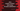# Create Random int,float, boolean using ThreadLocalRandom in JavaSimilar to ‘java.util.Random’ or ‘Math.random()’, ThreadLocalRandom is also a Random number generator in Java. With multithreading environment, it is better to use ‘ThreadLocalRandom’ instead of above two.

Using ‘ThreadLocalRandom’ in multithreaded environment will typically encounter much less overhead and contention. In this example, I will show you how to create a random number using ThreadLocalRandom :

To initialize a new ThreadLocalRandom, we need to call its ‘current’ method as below :

``ThreadLocalRandom random = ThreadLocalRandom.current();``

Now we can use this variable to create random values.

### Create a random value using ThreadLocalRandom :

After creating a ThreadLocalRandom object, we can easily produce a random value. Let’s take a look into some useful methods :

_next(int bits) : _Generates the next pseudorandom number.

nextBoolean() : Generates a pseudorandom boolean value

nextDouble() : Generates a pseudorandom double value between zero and one

nextDouble(double bound) : Generates a pseudorandom double value between zero and bound

nextDouble(double origin, double bound) : Generates a pseudorandom double value between origin and bound

nextFloat() : Generates a pseudorandom float value between zero and one

nextInt() : Generates a pseudorandom int

nextInt(int bound) : Generates a pseudorandom int value between zero and bound

nextInt(int origin, int bound) : Generates a pseudorandom int value between origin and bound

nextLong() : Generates a pseudorandom long

nextLong(long bound) : Generates a pseudorandom long value between zero and bound

nextInt(int origin, int bound) : Generates a pseudorandom long value between origin and bound

Following example explains each of these methods :

``````/*
*
* you may not use this file except in compliance with the License.
* You may obtain a copy of the License at
*
*
* Unless required by applicable law or agreed to in writing, software
* WITHOUT WARRANTIES OR CONDITIONS OF ANY KIND, either express or implied.
* See the License for the specific language governing permissions and
*/

/**
* Example class
*/
public class ExampleClass {

//utility method to print a string
static void print(String value) {
System.out.println(value);
}

public static void main(String[] args) {

//random boolean
print("Random boolean : "+random.nextBoolean());
print("-------------------------------------------------");

//random integer
print("Random int : "+random.nextInt());

print("Random int between 0 and 10 : "+random.nextInt(10));

print("Random int between 10 and 20 : "+random.nextInt(10,20));

print("-------------------------------------------------");

//random float
print("Random float : "+random.nextFloat());
print("-------------------------------------------------");

//random long
print("Random long : "+random.nextLong());

print("Random long between 0 and 10 : "+random.nextLong(10));

print("Random long between 10 and 20 : "+random.nextLong(10,20));

}

}``````

The output maybe different on your system. It will be similar like below :

``````Random boolean : false
-------------------------------------------------
Random int : -2127228988
Random int between 0 and 10 : 3
Random int between 10 and 20 : 12
-------------------------------------------------
Random float : 0.4902202
-------------------------------------------------
Random long : -5166997190540139296
Random long between 0 and 10 : 0
Random long between 10 and 20 : 17``````